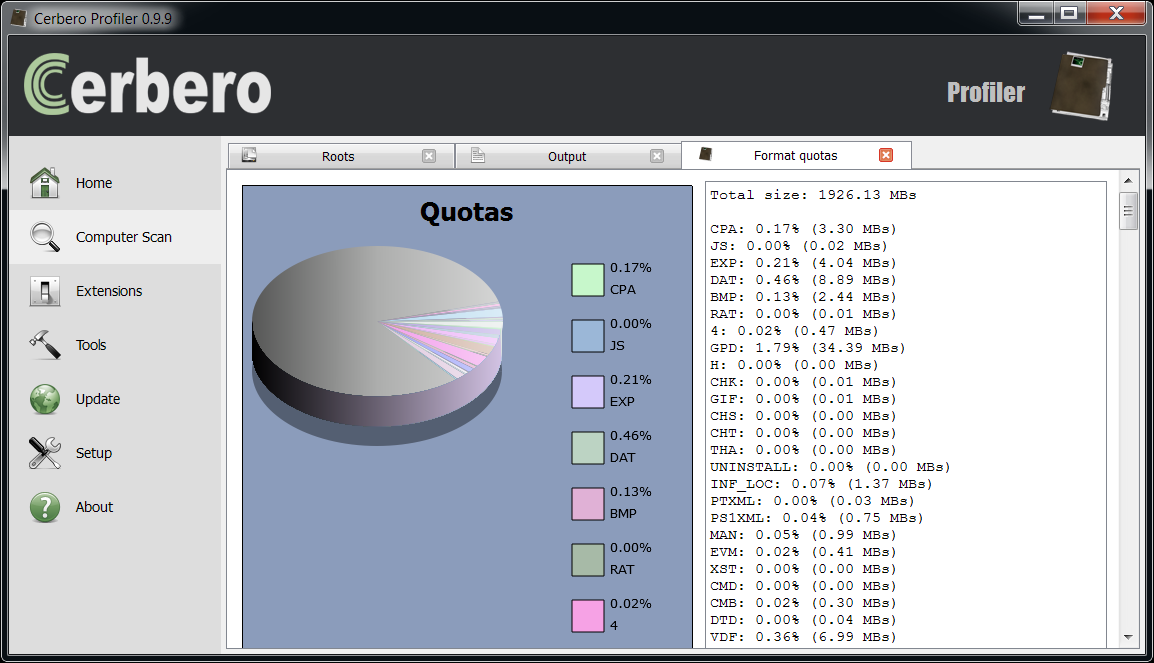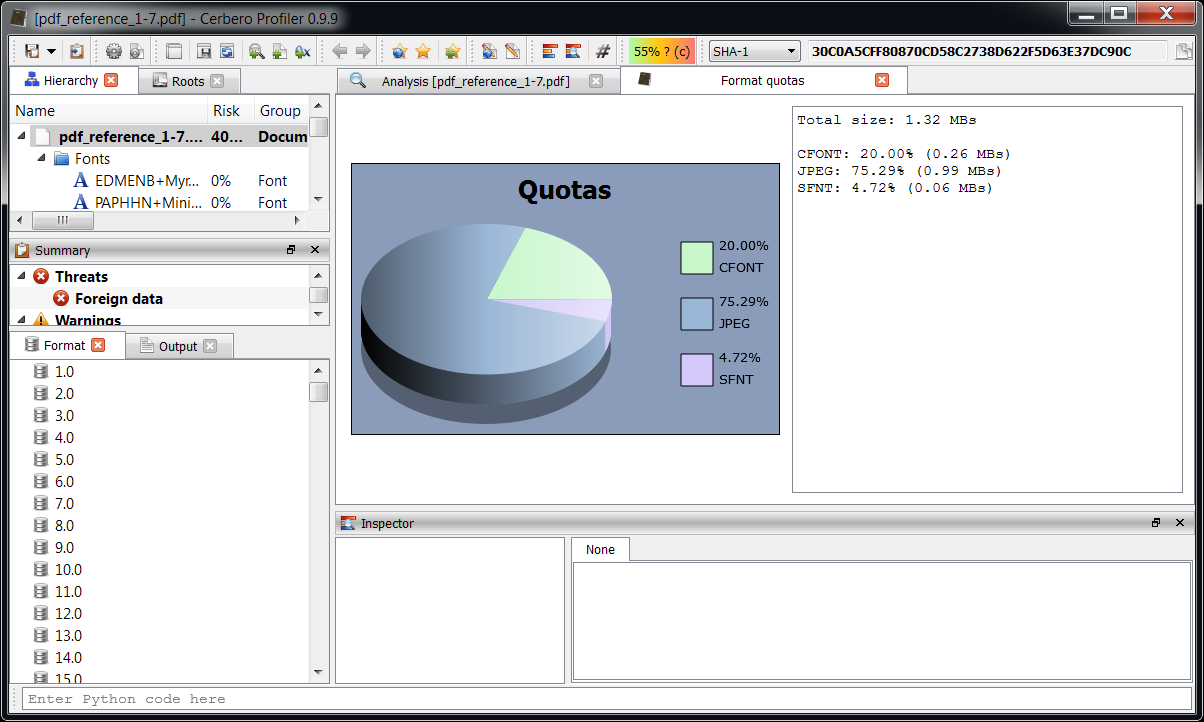## Format quota calculator

In the upcoming 0.9.9 version of the Profiler it will be possible to create docked views even in the context of the main window. This feature combined with custom views is extremely useful if we want to create custom reports at the end of a scan.

Some time ago I needed a little script to calculate the format quotas of files in a specific directory and their sub-files: we’ll use this sample to demonstrate the new features. For example we could use it to determine what kind of files and in what percentage the System32 directory on Windows contains. Or we could use it to determine the quotas of files in a Zip archive. To make it even more useful, the script now asks the user before the scan to enter the nesting range to consider. For example the value ‘0’ means all levels (starting from 0). If we want to calculate the quotas of top level files only, we must insert ‘0-0’ (start-end). The files contained in a Zip archive can be calculated with the value ‘1-1’ and if we want to include their sub-files we must insert ‘1’.We’re probably going to include the script in the upcoming release. But in case we don’t, in order to try it out, add the following lines to the hooks configuration file:

```[Format Quota Calculator]
file = quotas.py
init = typeQuotaCalcaulatorInit
end = typeQuotaCalcaulatorEnd
scanned = typeQuotaCalcaulatorScanned```

And create a ‘quotas.py’ file in your ‘plugins/python’ user directory with the following content:

```from os import path
import random

def generateColor():
c = ""
for i in range(3):
c = c + "%0.2X" % ((random.randint(0, 200) + 300) >> 1)
return c

def typeQuotaCalcaulatorInit():
random.seed(0)
# ask for nesting levels to consider
from Pro.UI import ProInput
ns = ProInput.askText("Format Quota Calculator (nesting level: from(-to))", "0")
lstart = 0
lend = -1
if ns != None:
ns = ns.split("-")
if len(ns) > 0:
lstart = int(ns)
if len(ns) > 1:
lend = int(ns)
return { "lstart" : lstart, "lend" : lend, "total" : 0, "quotas" : { } }

def typeQuotaCalcaulatorEnd(ud):
from Pro.UI import proContext, ProView
from html import escape
prec = "%.2f"
mbsize = 1024 * 1024
u = ud["total"] / 100
# prepare content
s = "Total size: " + (prec % (ud["total"] / mbsize)) + " MBs\n"
ui = ""
for k,q in ud["quotas"].items():
ps = (prec % (q / u))
ss = (prec % (q / mbsize))
s = s + "\n" + k + ": " + ps + "% (" + ss + " MBs)"
ui = ui + ""
ui = ui + ""
# display view
ctx = proContext()
v = ctx.createView(ProView.Type_Custom, "Format quotas")
v.setup(ui)
v.getView(1).setText(s)

def typeQuotaCalcaulatorScanned(sp, ud):
# check nesting
nesting = sp.scanNesting()
if ud["lstart"] > nesting or (ud["lend"] >= 0 and ud["lend"] < nesting):
return
c = sp.getObjectStream()
fmt = sp.getObjectFormat()
# if we didn't recognize the file, use extension as format identifier
# we could also use an external signature db...
if fmt == "":
fmt = path.splitext(c.name())
if len(fmt) > 0:
fmt = fmt[1:] # skip dot
if len(fmt) == 0:
fmt = "?"
else:
fmt = fmt.upper()
size = c.size()
ud["total"] = ud["total"] + size
if not fmt in ud["quotas"]:
ud["quotas"][fmt] = 0
ud["quotas"][fmt] = ud["quotas"][fmt] + size```

Remember to activate the hook from the UI before running a scan.

Of course, the view will be displayed even after an individual file scan in the workspace.In order to improve the script, we could use an external signature database for those file formats not recognized automatically.

This is a perfect example of the capabilities to extend the functionality of the Profiler. While there’s yet no estimated release date for the upcoming version, keep in tune as we hope to publish very interesting stuff soon.